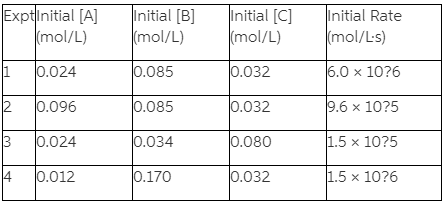# Problem: Consider the following general reaction and data:2A + 2B + C → D + 3E(a) What is the reaction order with respect to each reactant?(b) Calculate the rate constant.(c) Write the rate law for this reaction.

###### FREE Expert Solution

We’re being asked to calculate the rate law and the value of the rate constant (k) based on the reaction and data given.

Recall that the rate law only focuses on the reactant concentrations and has a general form of:

k = rate constant
A & B = reactants
x & y = reactant orders

Step 1. Calculate the order of the reaction with respect to [A].

[A]i (M)                   [B]I (M)                [C]I (M)                 Initial Rate (M-1 s-1)

1                     0.024                      0.085                    0.032                     6.0 x 10-6
2                     0.096                      0.085                    0.032                     9.6 x 10-5
3                     0.024                      0.034                    0.080                     1.5 x 10-5
4                     0.012                      0.170                    0.032                     1.5 x 10-6

Calculate order with respect to NO:

x = order

(Larger concentration should be on the numerator)

2 raised to the 2nd power = 4

x = 2 → 2nd order with respect to [A]

Step 2. Calculate the order of the reaction with respect to [B].

[A]i (M)                   [B]I (M)                [C]I (M)                 Initial Rate (M-1 s-1)

1                     0.024                      0.085                    0.032                     6.0 x 10-6
2                     0.096                      0.085                    0.032                     9.6 x 10-5
3                     0.024                      0.034                    0.080                     1.5 x 10-5
4                     0.012                      0.170                    0.032                     1.5 x 10-6

Calculate order with respect to [B]:

y = order

(Larger concentration should be on the numerator)

0.2 raised to the 0th power = 1

y = 0 → 0th order with respect to [B]

Step 3. Calculate the order of the reaction with respect to [C].

[A]i (M)                   [B]I (M)                [C]I (M)                 Initial Rate (M-1 s-1)

1                     0.024                      0.085                    0.032                     6.0 x 10-6
2                     0.096                      0.085                    0.032                     9.6 x 10-5
3                     0.024                      0.034                    0.080                     1.5 x 10-5
4                     0.012                      0.170                    0.032                     1.5 x 10-6

Calculate order with respect to [C]:

z = order

(Larger concentration should be on the numerator)

2.5 raised to the 1st power = 2.5

y = 1 → 1st order with respect to [C]

Step 4. Determine the rate law of the reaction

Substitute NO and O2 and their orders:

rate law = k[A]2[B]0[C]1

or simply

rate law = k[A]2[C]

The rate law is rate law = k[A]2[C]

83% (470 ratings)###### Problem Details

Consider the following general reaction and data:

2A + 2B + C → D + 3E(a) What is the reaction order with respect to each reactant?

(b) Calculate the rate constant.

(c) Write the rate law for this reaction.